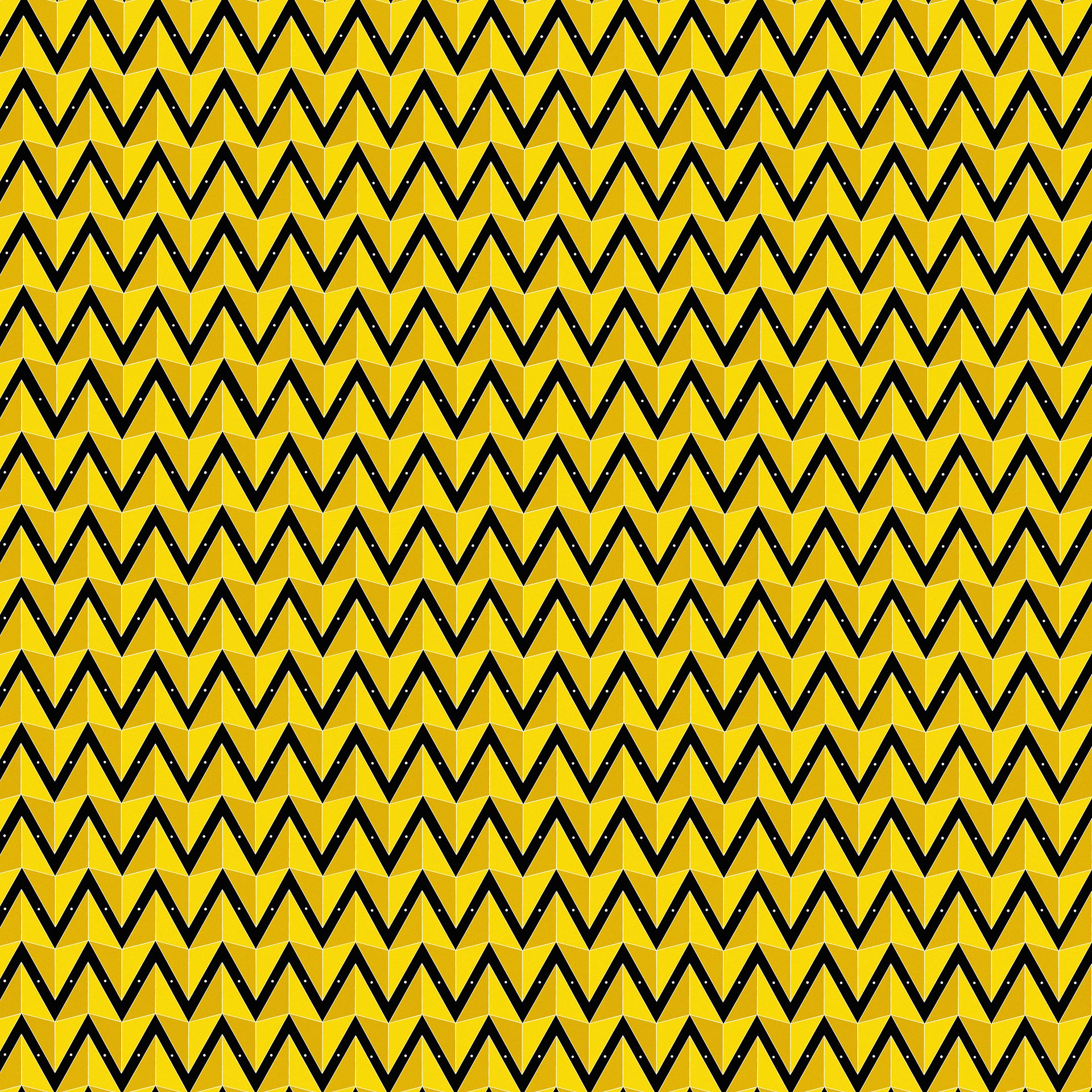top of page# The Law of Harmony

This post illustrates how I developed the "Master Key" to the keyboard.

The keyboard is a mathematical interpretation of sound based on harmonic ratios or intervals. An interval is a difference between two pitches or notes. All intervals hold a distinct quality, whether they are: perfect, major, minor, augmented, or diminished. There are four intervals known as perfect intervals: the unison (1:1), the octave (2:1), the perfect fifth (3:2), and the perfect fourth (4:3). While the unison interval is the same note repeated, and the octave is the same note with twice or half the frequency of vibration; the perfect fourth and the perfect fifth are a series of alternating notes that are known as the "Circle of Fourths" or the "Circle of Fifths." These two cycles are synonymous as they produce the arrangement of keys on the keyboard.

Intervals are the building blocks of music. Combining intervals create what are known as chords, which are the building blocks of harmony. The most fundamental chords are the major and minor triads because they contain only consonant intervals. Any other triad is considered a deviation from one of these two basic forms.The Major triad is constructed by a major third, a minor third, and a perfect fifth. Depicted left, for example, the space of three notes between the C and E (D flat, D, E flat) represents the major third. The space of two notes between the E and G is the minor third. The space of six notes between C and G is the perfect fifth.

Triangles are the strongest geometric building blocks. The rule of triangles states: the sum of any two sides must be greater than or equal to the third side (Ex.3+4 >2, 2+4 > 3, 3+2 > 4). The space of six units between C and G does not allow for a 3-2-6 triangle to exist. Instead, the six units between C4 and G4 can be substituted with the four units between G3 and C4 because the nature of the Circle of Fourths/Fifths is interchangeable. Once substituted it creates the 3-2-4 triangle representing the basic building block of harmony.Similar to the major triad, the minor triad has the same principles except that the major third and the minor third are switched. For example, the space of two notes between the A and C (B flat and B) is the minor third. The space of three notes between the C and E is the minor third. The space of six notes between A and E is the perfect fifth.

There is a major and minor triad for each of the twelve keys in an octave, 24 total. Each triad is connected by a set of pairs that form the natural connection of the keyboard.The First Relative Major and Minor are two triads that share the same major third. For example, depicted left, A minor and C major are paired along the 'three' side of the triangles. The arrowhead that they form is representative of the C major scale that is the key signature of these two specific triads.The Second Relative Major and Minor are linked by the minor third. Depicted left, C major pairs with E minor along the triangles' sides numbered 2. The major and minor triad in this pairing represents the link between adjacent scales. For example, the C major triad belongs to the key signature of C, and the E minor triad belongs to the adjacent key signature of G. Based on these pairings we can create the greater network of the keyboard.

All notes, chords, and scales are connected through this pairing.Based on the Circle of Fifths/Fourths, the respective key signatures can be applied.

Yellow triangles represent the root positions and non-yellow triangles represent inversions.Every triad has a root position and two inversions. Therefore, three layers underlie the keyboard; each layer is divisible by a major and a minor cycle, creating six cycles total.The layers are interchangeable.

The white dots represent polar opposites (overlapping major and minor triads).These layers form the complete geometric representation of the keyboard.Geometry is number in space. Sound, frequencies of vibration, is number in time.Space-Timebottom of page